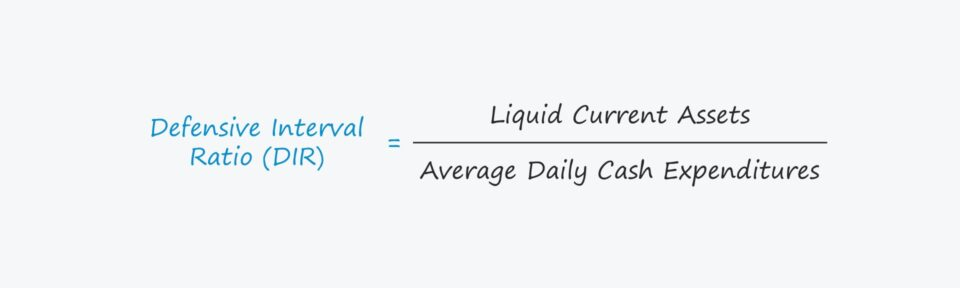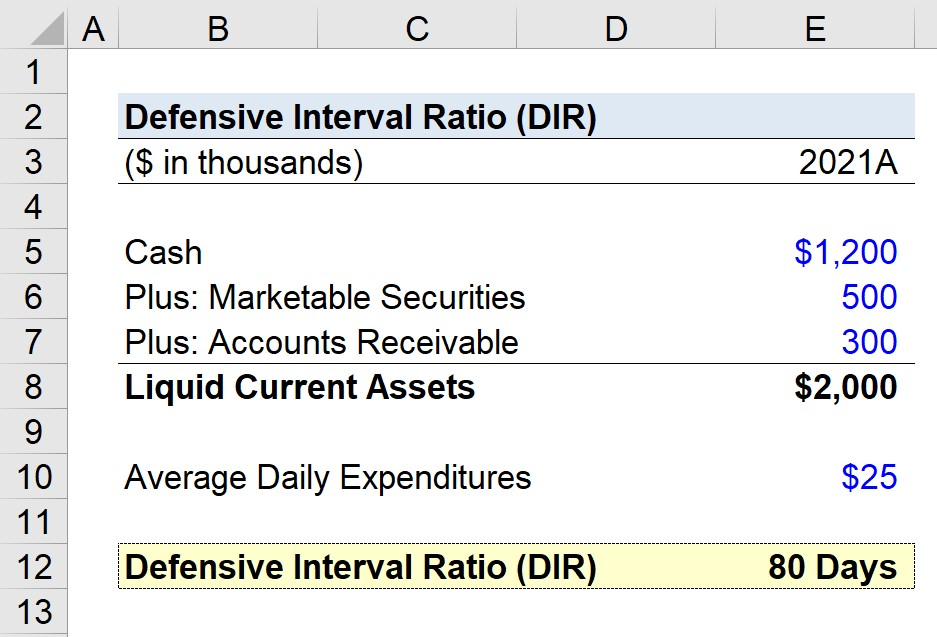# Defensive Interval Ratio (DIR)

Guide to Understanding the Defensive Interval Ratio (DIR)## How to Calculate the Defensive Interval Ratio

DIR stands for “defensive interval ratio” and is a tool for evaluating a company’s liquidity position.

The defensive interval ratio (DIR) estimates the number of days that a company can continue operating using only its liquid assets without seeking external financing or resorting to other methods to obtain cash such as attempting to sell its fixed assets.

The DIR is useful because it is particularly conservative, i.e. the factors considered are more stringent because only current assets with the highest liquidity are included.

Moreover, there is a limit to how much daily expenditures can be adjusted to appear more favorable, especially when expense reports are granular and based on recent monthly (or weekly) statements.

In contrast, there are other cash-flow-oriented measures of liquidity where management’s projections for profitability and free cash flow (FCF) can conceal the actual risk attributable to the company.

In order to calculate the equity ratio, there are three steps involved:

• Step 1 → Determine the Liquid Current Assets
• Step 2 → Estimate the Monthly Cash Expenses
• Step 3 → Divide the Sum of the Liquid Current Assets by the Monthly Cash Spend

## Defensive Interval Ratio Formula

The formula for calculating the defensive interval ratio is as follows.

###### Formula
• Defensive Interval Ratio (DIR) = Liquid Current Assets ÷ Average Daily Cash Expenditures

The term “Liquid Current Assets”, or quick assets, refer to current assets that can be converted into cash very quickly.

Further, the daily cash expenditures must represent actual cash outflows, as opposed to non-cash items such as depreciation or amortization.

## Defensive Interval Ratio Calculator – Excel Template

We’ll now move to a modeling exercise, which you can access by filling out the form below.Submitting ...

## DIR Calculation Example

Suppose we’re tasked with calculating the defensive interval ratio (DIR) for a company at the end of its latest fiscal year reporting period, 2021.

With 2021 now in the books, management wants to assess the liquidity of their company, or more specifically, how long the company could last if it relied solely on its liquid current assets (and no other external funding sources or non-current assets).

The following carrying values are found in the company’s latest 10-K.

• Cash = \$1.2 million
• Marketable Securities = \$500k
• Accounts Receivable = \$300k

Upon adding them together, the company’s total liquid current assets sum up to \$2 million.

As for the average daily expenditures – i.e. the amount of cash spent per day – we’ll assume the company spends \$25k each day.

In the final step of our exercise, we can calculate the defensive interval ratio (DIR) as 80 days by dividing the liquid current assets by the average daily expenditures.

• Defensive Interval Ratio (DIR) = \$2 million ÷ \$25k = 80 Days

This implies that our hypothetical company’s operations can continue running as normal for approximately 80 days if it were to rely only on its liquid current assets.Step-by-Step Online Course

### Everything You Need To Master Financial Modeling

Enroll in The Premium Package: Learn Financial Statement Modeling, DCF, M&A, LBO and Comps. The same training program used at top investment banks.